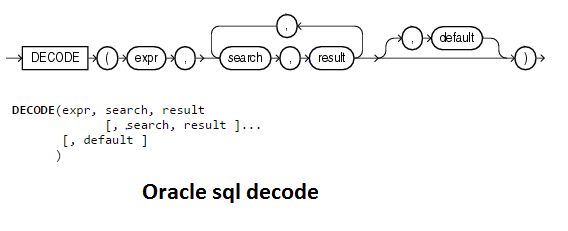# Oracle sql decode processing

Last updated on March 9th, 2019 at 04:33 am

## Introduction to Oracle sql decode

In this section we will discuss about Oracle decode processing which is very important aspect in Oracle sql statement

Oracle  decode is the method in Oracle database to  transform data values for one value to another which is better to understand.Oracle Decode transform data values at retrieval time.It is a type of If then else for the processing

The code block for Oracle sql Decode is below

decode(expression or column name, match, result [,match, result]…[,default] )

Here are the meaning for terms in above code
a) expression or column  is the value to compare
b) match is the value that is compared against expression
c) result is the value returned, if expression is equal to match
d) default is optional. If no matches are found, the decode will return default. If default is omitted, then the decode statement will return NULL (no matches found).

 Example select decode ( Phase Code, ‘P’,’Pending’, ‘C’,’Completed’, ‘T’,’Terminated’, ‘S’,’Standby’, ‘UNKNOWN’ ) from FND_REQUESTS;

Here is the algorithm to better understand it

1) Oracle retrieve  the column value of Phase code
2) if Phase code = ‘P’  then Pending
3) if Phase code = ‘C’  then Completed
4) if Phase code = ‘T’  then Terminated
5) if Phase code = ‘S’  then Standby
6) If Phase code is neither of the above ,the decode returns Unknown
7) If default is not present it will give null

Note that Oracle decode starts by specifying the column name or expression , followed by set of matched-pairs of transformation values. At the end of the decode statement we find a default value. The default value tells decode what to display if a column values is not in the paired list.

We could say the algorithm like this way

 if (expr == search1) return(result1); elseif (expr == search2) return(result2); …elseif (expr == searchn) return(resultn); else return(default);

## Some More points to remember for Oracle Decode

1)In a DECODE function, Oracle considers two nulls to be equivalent. If expr is null, then Oracle returns the result of the first search that is also null.

SQL> SELECT decode(null,null,1,0) FROM dual;
DECODE(NULL,NULL,1,0)
———————
1

2) The maximum number of components in the DECODE function, including expr, searches, results, and default, is 255.

3)Oracle automatically converts the values for expression and compare_value to the datatype of the first compare_value. Also the datatype of the return_value is converted to the datatype of the first return_value. If the first result has the datatype CHAR or if the first result is null, then Oracle converts the return value to the datatype VARCHAR2

## FAQ about Oracle sql Decode processing

1.  We have seen that expr being equated to specified values,Can we use unequality operator like > or <  ?

Answer

Lets take an example

 SELECT DECODE(salary,< 50000, sal + 1000, sal + 500) Final_salary FROM emp; ERROR at line 2: ORA-00936: missing expression

So we cannot use that. We need to use case statement to do it.  or we can sign function in decode  to achieve it

 SELECT DECODE(sign(salary- 50000), -1,sal + 1000, sal + 500) Final_salary FROM emp;

Basically we need to convert our requirement to some formula which can evaluate to some value

2) How to compare two column values using oracle decode?

Answer

 SELECT col1,col2 decode( abs(col1-col2), 0, ‘col1 = col2’,Col1-col2, ‘col1 > col2′,’col1 < col2’) FROM example_tab;

Related links

Oracle documentation on decode

Oracle sql and plsql

how to write sql queries

Oracle sql tutorial :Basic Sql statement

Oracle Case Statement Explained with Tips and Examples